• 在平面直角坐标系中，怎么确定唯一的一条直线？答案是：直线上的一个定点以及它的倾斜角，二者缺一不可。坡度(图1)生活中使用用“升高量与前进量的比”表示...我们把一条直线的倾斜角a的正切值叫这条直线的斜率(slo...
在平面直角坐标系中，怎么确定唯一的一条直线？答案是：直线上的一个定点以及它的倾斜角，二者缺一不可。坡度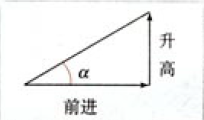(图1)生活中使用用“升高量与前进量的比”表示倾斜面的“坡度”，即：坡度(比)=升高量/前进量例如，“进3升3”，“进2升2”，指的坡度比为分别为：  3/2， 2/2，前者改陡一些。斜率如图1，坡度比实际上就是tan(a)，即倾斜角的正切。我们把一条直线的倾斜角a的正切值叫这条直线的斜率(slope)。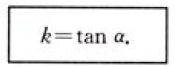给定两点的斜率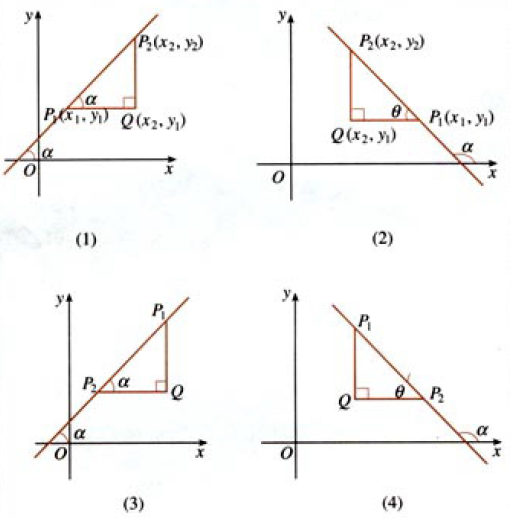(图2)图2-(1)的斜率为：k=tan a=|QP2|/|QP1|=(y2-y1)/(x2-x1)图2-(2)的斜率为：tan a=tan(180°-θ)=-tanθtanθ=|QP2|/|QP1|=-((y2-y1)/(x1-x2))于是可得：k=tan a=(y2-y1)/(x1-x2)图2-(3)的斜率为：注意这里是P2P1的方向向上时，也有：k=tan a=(y2-y1)/(x2-x1)综上所述，我们得到经过两点P1(x1,y1), P2(x2,y2) (x1≠x2)的直线斜率的公式：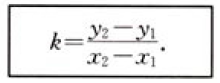例题题目1：如下图3，已知A(3,2)，B(-4,1), C(0,-1)，求直线AB，BC，CA的斜率，并判断这些直线的倾斜角是锐角还是钝角。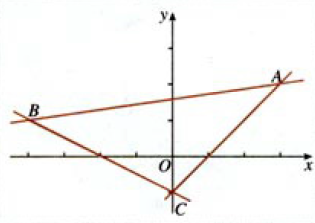(图3)解：注意按向上方向确定是那个点是y2/x2KAB=(1-2)/(-4-3)=1/7KBC=(-1-1)/(0-(-4))=-2/4=-(1/2)KCA=(-1-2)/(0-3)=-3/-3=1因为KAB>0和KCA>0，所以直线AB，CA的倾斜角为锐角。因为KBC<0，所以直线BC的倾斜角为钝角。斜率的应用(1)平等关系判定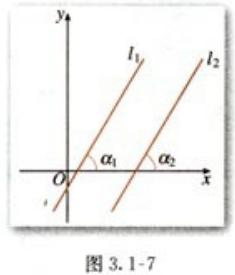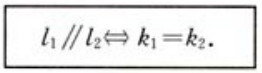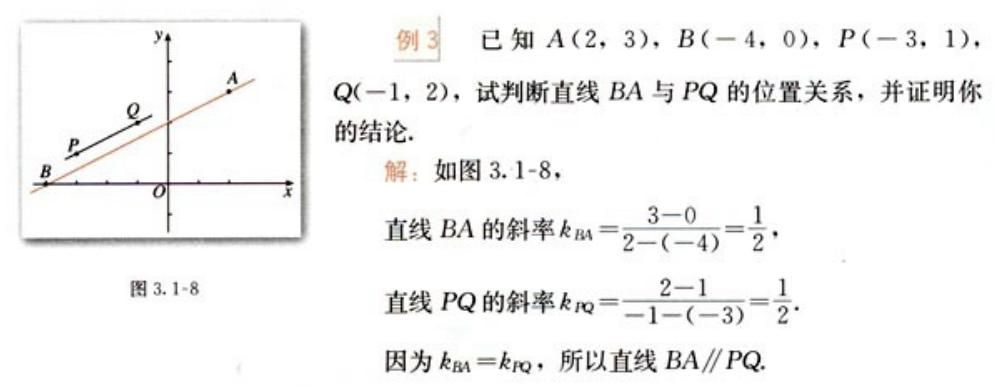(2)垂直关系判定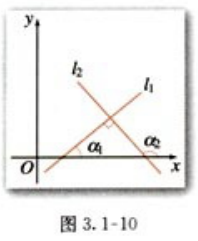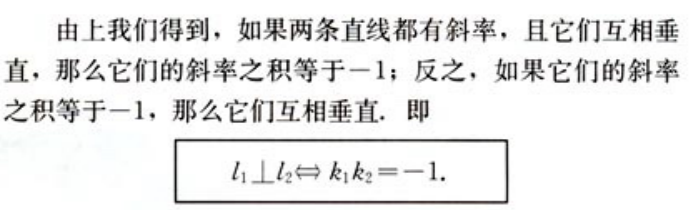例题：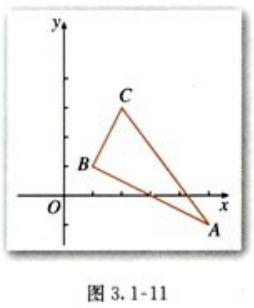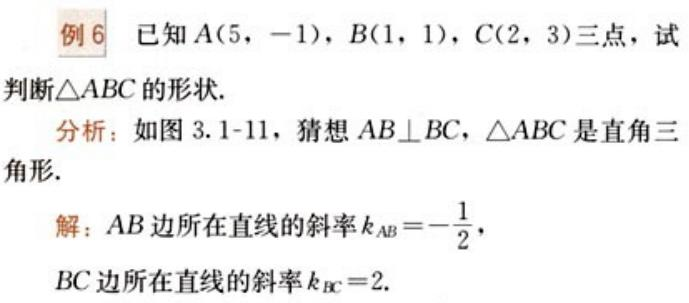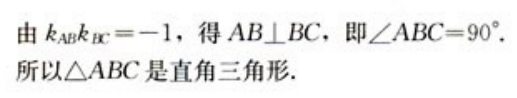---------------------作者：hackpig来源：www.skcircle.com版权声明：本文为博主原创文章，转载请附上博文链接！#转载请注明出处 www.skcircle.com 《少有人走的路》勇哥的工业自动化技术网站。如果需要本贴图片源码等资源，请向勇哥索取。收藏 | 0点赞 | 0打赏作者
展开全文• 文档图像倾斜角检测及校正（三）； 斜率空间投票原理； Matlab程序； 阅读之前注意： Hi，你好，我是Cooper Liu，欢迎来到我写的“文档图像校正”系列博客。基于三种原理，我写了四个实验...
文档图像倾斜角检测及校正（三）斜率空间投票原理
Matlab程序
阅读之前注意：

Hi，你好，我是Cooper Liu，欢迎来到我写的“文档图像校正”系列博客。基于三种原理，我写了四个实验性的Matlab验证程序，以及两个文档校正Matlab程序。在这里你将能够获取所有的源代码以及测试图片，完全可以在你自己的Matlab上跑这些程序。
如果你是学生，请注意不要抄袭，课程设计作业的话，这种程序仅仅只能让你得到80%左右的成绩。
如果你是工作人士或者只是感兴趣的极客，Okay，我想这些程序对于理解原理是如何应用为程序的已经足够 。
最后，请勿将这些资源用于商业用途（如你所见，这些程序都非常的初级）或者是谋取个人利益，知识在传播的过程中能展现更大的价值-

本文阅读建议用时：42min
本文阅读结构如下表：

项目
下属项目
测试用例数量
斜率空间投票原理
无
0
Matlab程序
无
2
斜率空间投票原理1
斜率空间投票指的是，在文档图像的某一个区域中，如果我们知道有一个点(x0,y0)(x_0,y_0 )是直线上的点，那我们将该点所在的直线斜率定为m，则坐标和斜率的关系可表示为y0=m(x−x0)y_0=m(x-x_0)。由此图像区域中的每个目标像素(x′,y′)(x',y')与(x0,y0)(x_0,y_0 )点之间连线的斜率m′m'可表示为m′=(y′−y0)/(x′−x0)m'=(y'-y_0)/(x'-x_0)。
我们将斜率值映射到一组累加器上，每求出一个斜率值，对应的累加器加一。因为同一直线上的点求得的斜率相同，所以累加器会出现局部最大值，该值所对应的斜率即是所求直线的斜率，倾斜角也能相应的求出。
值得注意的是，当x′=x0x'=x_0时，m′m'为无穷大。为了避免这一情况，当x′=x0x'=x_0时，我们令m′=2m'=2，而当m′>1m'>1或m′<1m'<1时，我们采用公式m′′=1/m′−2m''=1/m'-2的计算值代替m′m'。这样一来，m′m'的范围即被限定在(-1,3)的区间内。
Matlab程序
基于以上原理，我编写了Matlab程序来进行实验，源代码可以参考angleDetection3.m文件和angleDetection4.m文件。测试图片为line5.bmp。2
测试图片可以从这里获取，链接：https://pan.baidu.com/s/1dGmmGjn 密码：okt3
我编写了两个版本的Matlab程序，其中版本一（angleDetection3.m文件）的思想是把斜率区间尽可能的细分，比如分成10000份，再计算对应10000个累加器的最大值，从而反推出斜率。而版本二（angleDetection4.m文件）的思想则是先把斜率空间分为10个子区间，求出累加器的最大值，把最大值对应的子区间再分为10个子区间，如此迭代，直至区间宽度小于我们所控制的迭代精度。
以下是版本一的实验结果：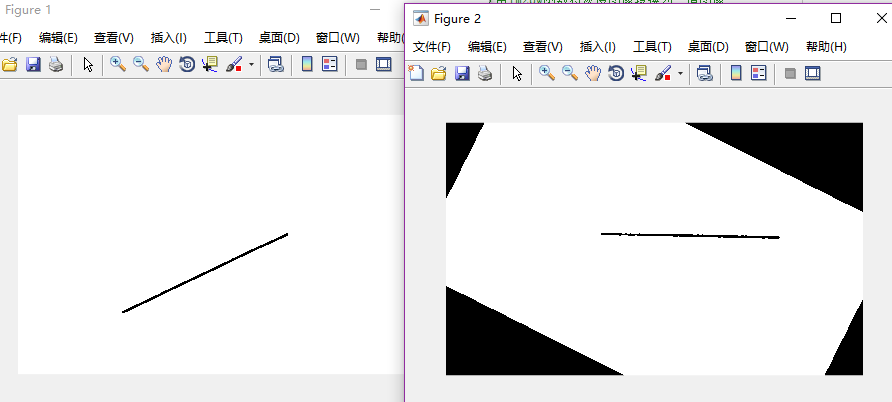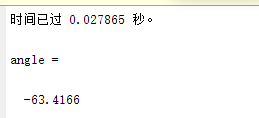以下是版本二的实验结果：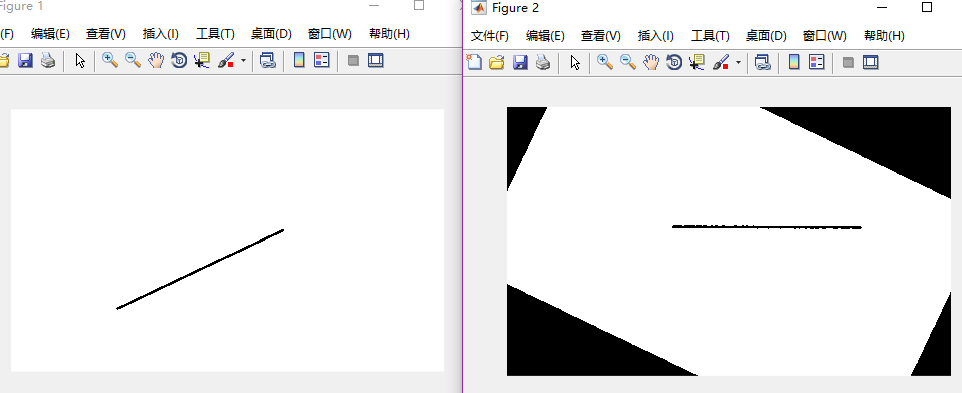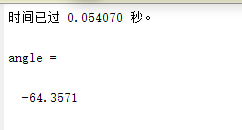如果您不想打开新的页面查看matlab源代码，也可以直接参考以下代码：
版本一（angleDetection3.m文件）：
%%本版基于斜率空间投票原理
%%2018.01.20_14:09 by Cooper Liu
%%Questions? Contact me: angelpoint@foxmail.com
clear;clc; %清空之前的变量
level=graythresh(I); %使用最大类间方差法找到图片的一个合适的阈值
bw=im2bw(I,level); %根据阈值，使用im2bw函数将灰度图像转换为二值图像
figure(1);imshow(bw);
[m,n]=size(bw); %获取尺寸
flag=0;
tic; %计时开始
for i=1:m
for j=1:n
if bw(i,j)==0
if flag==0
x0=i;y0=j; %获取第一个点
flag=1;
end
end
end
end
range=10000; %range越大对角度分得更细，计算量更大
low=-1;
high=3;

a=zeros(range,1);
for i=1:m
for j=1:n
if bw(i,j)==0
if i==x0
k=2;
area=floor((k-(-1))/4*range); %向下取整
a(area+1,1)=a(area+1,1)+1; %区间计数
else
k=(j-y0)/(i-x0);
k2=k;
if  k>1 || k<-1
k2=2-1/k; %转换斜率，保证斜率范围（-1, 3）
end
area=floor((k2-(-1))/4*range); %向下取整
a(area+1,1)=a(area+1,1)+1;
end
end
end
end
toc %获取时间
[s_i,index] = sort(a,'descend'); %降序排序并获取原来序列的序号至index
max=index(1);
tmp=(-1)+max/range*4;
if tmp>1
k=1/(2-tmp); %还原原来的斜率
else
k=tmp;
end
angle=atan(k); %用atan求出来的角度在-pi/2到+pi/2之间
angle=angle*180/pi

rot=90-angle;
pic=imrotate(I,rot,'crop'); %旋转图像
figure(2);imshow(pic);

版本二（angleDetection4.m文件）：
%%本版基于斜率空间投票原理，改进自angleDetection3.m
%%2018.01.20_15:41 by Cooper Liu
%%Questions? Contact me: angelpoint@foxmail.com
clear;clc; %清空之前的变量
level=graythresh(I); %使用最大类间方差法找到图片的一个合适的阈值
bw=im2bw(I,level); %根据阈值，使用im2bw函数将灰度图像转换为二值图像时
figure(1);imshow(bw);
[m,n]=size(bw); %获取尺寸
flag=0;

for i=1:m
for j=1:n
if bw(i,j)==0
if flag==0
x0=i;y0=j;
flag=1;
end
end
end
end
range=10; %整个斜率区间分为10个子区间
low=-1;
high=3;
tmpLow=low;
tmpHigh=high;
max=0;
tic; %开始计时
while high-low>0.0001
a=zeros(range,1);
for i=1:m
for j=1:n
if bw(i,j)==0
if i==x0 %如果x坐标与x0相同
k=2; %则固定k为2
if k>=low && k<=high
area=floor((k-low)/(high-low)*range); %向下取整
if area==range %如果等于最后一个区间
area=area-1; %请考虑到后面区间计数的area+1
end
a(area+1,1)=a(area+1,1)+1; %区间计数
end
else     %如果x坐标与x0不同
k=(j-y0)/(i-x0); %则计算斜率
k2=k;
if  k>1 || k<-1
k2=2-1/k;
end
if k2>=low && k2<=high
area=floor((k2-low)/(high-low)*range); %向下取整
if area==range
area=area-1;
end
a(area+1,1)=a(area+1,1)+1;
end
end
end
end
end
[s_i,index] = sort(a,'descend');
max = index(1);
tmpLow = low;
tmpHigh = high;
if max>=2 && max<=9
else
end
low = tmpLow + (max - 1 - areaAddition)/range*(tmpHigh - tmpLow);
high = tmpLow + (max + areaAddtion)/range*(tmpHigh - tmpLow);
end

toc %获取计时时间

tmp=tmpLow+max/range*(tmpHigh-tmpLow);
if tmp>1
k=1/(2-tmp);
else
k=tmp;
end
angle=atan(k); %用atan求出来的角度在-pi/2到+pi/2之间
angle=angle*180/pi

rot=90-angle;
pic=imrotate(I,rot,'crop'); %旋转图像
figure(2);imshow(pic);

如果本文有帮助到你，不如请我一罐可乐吧 🎏文章目录文档图像倾斜角检测及校正（三）阅读之前注意：斜率空间投票原理[^1]Matlab程序
参考文献： 荆雷，张欣，郭金鑫．基于版面的拍照文档图像倾斜校正.激光与红外[J]．2010，第10期 ↩︎

同时感谢愿意在网络上分享自己想法的各位博主。 ↩︎


展开全文Matlab 数字图像处理
• 我们在写科赫雪花程序中，求顶点是一个难点，因为一般是要考虑不同斜率时顶点的不同情况。如果起始直线是水平的，那通过作图分析，还是很容易编写顶点的程序的： if ((x3&lt;x4)&amp;&amp;(y3==y4...

注意这篇文章不是研究科赫雪花的生成原理，而是讨论一个实现科赫雪花的更优的算法，关于分形的一些总结以后再说吧。当然这种思想也可以用于其他分形的程序，只要有足够的思考。我们在写科赫雪花程序中，求顶点是一个难点，因为一般是要考虑不同斜率时顶点的不同情况。如果起始直线是水平的，那通过作图分析，还是很容易编写顶点的程序的：
if ((x3<x4)&&(y3==y4)) {
x5 = (x3 + x4) / 2.0;
y5 = y3 - (x4 - x3) * Math.sqrt(3.0) / 2.0;
}
else if ((x3<x4)&&(y3<y4)) {
x5 = x4 + (x4 - x3);
y5 = y3;
}
else if ((x3>x4)&&(y3<y4)) {
x5 = x4 + 2*(x3 - x4);
y5 = y4;
}
else if ((x3>x4)&&(y3==y4)) {
x5 = (x3 + x4) / 2.0;
y5 = y3 + (x3 - x4) * Math.sqrt(3.0) / 2.0;
}
else if ((x3>x4)&&(y3>y4)) {
x5 = x4 - (x3 - x4);
y5 = y3;
}
else if ((x3<x4)&&(y3>y4)) {
x5 = x3 - (x4 - x3);
y5 = y4;
}  
当然我这种写法是比较丑的，因为把0~360°的六种情况全写出来了，其实如果设k=(x3-x4)/(y3-y4),通过k的正负来判断倾斜方向可以让程序更简洁一点。 不过，这种投机的分析方法只能用在直线是水平的这一特殊的情况，如果直线是任意倾斜角度的，那就必须通过计算旋转角找顶点这种强硬的数学方法，程序编写的难度骤然上升。于是，有没有方法可以只通过两个三等分点的坐标通过简单的计算就可以求出所有情况的科赫雪花的顶点呢？答案是肯定的，下面我来介绍。
用三等分点坐标求任意倾斜角的科赫雪花顶点。
首先我给出这种方法的数学证明：如下图所示：直线的倾斜角是任意的，BC是确定线段上的三等分点，也就是B(x3,y3)和C(x4,y4)，这里把原直线忽略了。顶点是A，在科赫雪花的程序中通常就是(x5,y5)。这里作几条辅助线：过B点作一条水平的直线，过C作一条垂直的直线，相交于G，过A点作BC的高，过A点作垂直的直线交BC于F，过D作AF的垂线，垂足为E。为什么要这么作辅助线？因为既然要通过简单的计算得到结果，那充分利用B(x3,y3)和C(x4,y4)只通过加减法就可以得到的数据，比如CG的长度就是y4-y3，BG的长度就是x4-x3；另外就是要考虑顶点的位置，这里可以看到顶点A的坐标可以又D的坐标得出，D的坐标很好算，就是B、C的中点，因此接下来的任务就是通过三角形的关系找出DE和AE的长度。
首先容易证明：△BCG∽△AFD∽△DFE
而GC=y4-y3，x5=xE=(x3+x4)/2-DE 所以：x5=(x3+x4)/2+(y3-y4) *√3/2 （3）
这就是科赫雪花顶点的横坐标公式，适用于任意倾斜角的雪花。如果直线是右倾斜的，那么y3-y4将变成正数，顶点坐标将出现在中点坐标的右方，一样成立。
同样我们可以证明y5=(y3+y4)/2+(x4-x3)*√3/2 （4）
（3）、（4）式的好处在于写科赫雪花程序时无需再考虑倾斜情况，即使起始直线是20°，这个公式也能求出此时雪花顶点的位置，那么程序就简单多了：
void kochsnow(double x1,double y1,double x2,double y2,int depth,Graphics g){
//注意depth请在主函数中自行定义
if(depth<=1){
//完成递归的条件
g.drawLine((int)x1, (int)y1, (int)x2, (int)y2);
}else{
//三等分点坐标
double x3=x1*2/3 + x2*1/3;
double y3=y1*2/3 + y2*1/3;
double x4=x1*1/3 + x2*2/3;
double y4=y1*1/3 + y2*2/3;
//顶点坐标
double x5=(x3+x4)/2+(y3-y4)*Math.sqrt (3)/2;
double y5=(y3+y4)/2+(x4-x3)*Math.sqrt (3)/2;
//连接所有点，递归
kochsnow(x1,y1,x3,y3,depth-1,g);
kochsnow(x3,y3,x5,y5,depth-1,g);
kochsnow(x5,y5,x4,y4,depth-1,g);
kochsnow(x4,y4,x2,y2,depth-1,g);
}
}
这里我们可以优化这个程序：在画板上任意点两个点，行程一片科赫雪花，起始是正三角形，以后每点击一次科赫雪花的层数增加1，完整参考代码如下：

import java.applet.*;
import java.awt.*;
import java.awt.event.*;

public class Koch extends Applet implements MouseListener  {
private int xa,ya,xb,yb,depth,count=0;
private double xc,yc;

public void init()  {
setBackground(Color.BLACK);
setSize(960, 720);
depth=1;
}

public void mouseClicked(MouseEvent e) {}
public void mouseEntered(MouseEvent e) {}
public void mouseExited(MouseEvent e) {}
public void mousePressed(MouseEvent e){}
public void mouseReleased(MouseEvent e) {
depth++;
if(count==0){
xa = e.getX();
ya = e.getY();
count++;
depth=0;
}else if(count==1){
xb = e.getX();
yb = e.getY();
xc=(xa+xb)/2+(ya-yb)*Math.sqrt (3)/2;
yc=(ya+yb)/2+(xb-xa)*Math.sqrt (3)/2;
count=1;
repaint();
}
}

void draw(double x1,double y1,double x2,double y2,int depth,Graphics g){
if(depth<=1){
g.drawLine((int)x1, (int)y1, (int)x2, (int)y2);
}else{
depth--;
double x3=x1*2/3 + x2*1/3;
double y3=y1*2/3 + y2*1/3;
double x4=x1*1/3 + x2*2/3;
double y4=y1*1/3 + y2*2/3;
double x5=(x3+x4)/2+(y3-y4)*Math.sqrt (3)/2;
double y5=(y3+y4)/2+(x4-x3)*Math.sqrt (3)/2;
draw(x1,y1,x3,y3,depth,g);
draw(x4,y4,x2,y2,depth,g);
draw(x3,y3,x5,y5,depth,g);
draw(x5,y5,x4,y4,depth,g);
}
}

public void paint(Graphics g){
g.setColor(Color.WHITE);
draw(xb, yb, xa, ya, depth, g);
draw(xa, ya, xc, yc, depth, g);
draw(xc, yc, xb, yb, depth, g);
}
}

代码说明：
1. 这个程序的设计是点击两次后形成一个正三角形，然后每点击一次会重新找一个顶点，以便于调整三角形的大小来观察科赫雪花的变化，如果不想要让原来的三角形变化那就不要移动鼠标就行了。
2. 程序还可做进一步优化：左键点击深度+1，右键点击深度-1，这个我没写。
3. 这个程序没用面板，用的是applet，不过其实无所谓，核心算法在draw函数里，而且除了x5和y5也没特别厉害的地方，复制参考的话只要把那个函数复制了即可。

展开全文• 目前建设一个太阳能发电系统的成本还是较高的，从我国现阶段的太阳能发电成本来看，其花费在太阳电池组件的费用大约为30～40%，因此，为了更加充分有效地利用太阳能，如何选取太阳电池方阵的方位角与倾斜角是一个...


由于太阳能是一种清洁的能源，它的应用正在世界范围内快速地增长。利用太阳光发电就是一种使用太阳能的方式，目前建设一个太阳能发电系统的成本还是较高的，从我国现阶段的太阳能发电成本来看，其花费在太阳电池组件的费用大约为30～40%，因此，为了更加充分有效地利用太阳能，如何选取太阳电池方阵的方位角与倾斜角是一个十分重要的问题。今天笔者总结了几个增加发电量的方法。
1、方位角
太阳电池方阵的方位角是方阵的垂直面与正南方向的夹角(向东偏设定为负角度，向西偏设定为正角度)。一般情况下，方阵朝向正南(即方阵垂直面与正南的夹角为0°)时，太阳电池发电量是最大的。在偏离正南(北半球)30°度时，方阵的发电量将减少约10%～15%；在偏离正南(北半球)60°时，方阵的发电量将减少约20%～30%。
但是，在晴朗的夏天，太阳辐射能量的最大时刻是在中午稍后，因此方阵的方位稍微向西偏一些时，在午后时刻可获得最大发电功率。在不同的季节，太阳电池方阵的方位稍微向东或西一些都有获得发电量最大的时候。方阵设置场所受到许多条件的制约，例如，在地面上设置时土地的方位角、在屋顶上设置时屋顶的方位角，或者是为了躲避太阳阴影时的方位角，以及布置规划、发电效率、设计规划、建设目的等许多因素都有关系。如果要将方位角调整到在一天中负荷的峰值时刻与发电峰值时刻一致时，请参考下述的公式。至于并网发电的场合，希望综合考虑以上各方面的情况来选定方位角。
方位角=(一天中负荷的峰值时刻(24小时制)-12)×15+(经度-116)
10月9日北京的太阳电池方阵处于不同方位角时，日射量与时间推移的关系曲线。在不同的季节，各个方位的日射量峰值产生时刻是不一样的。
2、倾斜角
倾斜角是太阳电池方阵平面与水平地面的夹角，并希望此夹角是方阵一年中发电量为最大时的最佳倾斜角度。一年中的最佳倾斜角与当地的地理纬度有关，当纬度较高时，相应的倾斜角也大。但是，和方位角一样，在设计中也要考虑到屋顶的倾斜角及积雪滑落的倾斜角(斜率大于50%-60%)等方面的限制条件。对于积雪滑落的倾斜角，即使在积雪期发电量少而年总发电量也存在增加的情况，因此，特别是在并网发电的系统中，并不一定优先考虑积雪的滑落，此外，还要进一步考虑其它因素。对于正南(方位角为0°度)，倾斜角从水平(倾斜角为0°度)开始逐渐向最佳的倾斜角过渡时，其日射量不断增加直到最大值，然后再增加倾斜角其日射量不断减少。特别是在倾斜角大于50°～60°以后，日射量急剧下降，直至到最后的垂直放置时，发电量下降到最小。方阵从垂直放置到10°～20°的倾斜放置都有实际的例子。
对于方位角不为0°度的情况，斜面日射量的值普遍偏低，最大日射量的值是在与水平面接近的倾斜角度附近。以上所述为方位角、倾斜角与发电量之间的关系，对于具体设计某一个方阵的方位角和倾斜角还应综合地进一步同实际情况结合起来考虑。
太阳能电池组件的方位角与倾斜角选定是太阳能光伏系统设计时最重要的因素之一。所谓方位角一般是指东西南北方向的角度。对于太阳能光伏系统来说，方位角以正南为0°，由南向东向北为负角度，由南向本向北为下角度，如太阳在下东方向时，方位角为-90°，在正西方时方位角为90°。
方位角决定了阳光的入射方向，决定了各个方向的山坡或不同朝向的建筑物的采光状况。倾斜角是地平面(水平面)与太阳能电池组件之间的夹角。倾斜角为0°时表示太阳能电池组件为水平设置，倾斜角为90°时表示太阳能电池组件为垂直设置。
太阳能电池方位角的选择
在我国，太阳能电池的方位角一般都选择正南方向，以使太阳能电池单位容量的发电量最大。如果受太阳能电池设置场所如屋顶、土坡、山地、建筑物结构及阴影等的限制时，则应考虑与它们的方位角一到，以求充分利用现有的地形和有效面积，并尽量避开周围建、构筑物或树木等产生的阴影。
只要在正南±20°之内，都不会对发电量有太大影响，条件允许的话，应尽可能偏西南20°之内，使太阳能发电量的峰值出现在中午稍过后某时，这样有利冬季多发电。有些太阳能光伏建筑一体化发电系统设计时，当正南方向太阳能电池铺设面积不够大时，也可将太阳能电池铺设在正东、正西方向。太阳能电池倾斜角的选择
最理想的倾斜角是使太阳能电池年发电量尽可能大，而冬季和夏季发电量差异尽可能小时的倾斜角。一般取当地纬度或当地纬度加上几度做为当地太阳能电池组件安装的倾斜角。当然如果能够采用计算机辅助设计软件，可以进行太阳能倾斜角的优化计算，使两者能够兼顾就更好了，这对于高纬度地区尤为重要。
高纬度地区的冬季和夏季水平面太阳辐射量差异非常大，例如我国黑龙江省相差约5倍。如果按照水平面辐射量参数时行设计，则蓄电池冬季存储量过大，造成蓄电池的设计容量和投资都加大。选择了最佳倾斜角，太阳能电池面上冬季和夏季辐射量之差变小，蓄电池的容量也可以减少，求得一个均衡，使系统造价降低，设计更为合理。
如果没有条件对倾斜角进行计算机优化设计，也可以根据当地纬度粗略确定太阳能电池的倾斜角：
纬度为0°~25°时，倾斜角等于纬度；
纬度为26°~40°时，倾斜角等于纬度加上5°~10°；
纬度为41°~55°时，倾斜角等于纬度加上10°~15°；
纬度为55°以上时，倾斜角等于纬度加上15°~20°。
不同类型的太阳能光伏发电系统，其最佳安装倾斜角是有所不同的。例如光控太阳能路灯照明系统等季节性负载供电的光伏发电系统，这类负载的工作时间随着季节而变化，其特点是以自然光线的来决定负载每天工作时间的长短。
冬天时日照时间短，太阳能辐射能量小，而夜间负载工作时间长，耗电量大。因此系统设计时要考虑照顾冬天，按冬天时能得到最大发电量的倾斜角确定，其倾斜角应该比当地纬度角度大一些。而对于主要为光伏水泵、制冷空调等夏季负载供电的光伏系统，则应考虑为夏季负载提供最大发电量，其倾斜角应该比当地纬度的角度小一些。

本文转自d1net（转载）


展开全文• 在数学上，直线的斜率任何一处皆相等，它是直线的倾斜程度的量度。透过代数和几何，可以计算出直线的斜率；曲线上某点的切线斜率则反映了此曲线的变量在此点处的变化的快慢程度。运用微积分可计算出曲线中的任一点的...数学
• 斜率，亦称"系数"，表示一条直线相对于横轴的倾斜程度。一条直线与某平面直角坐标系横轴正半轴方向的夹角的正切值即该直线相对于该坐标系的斜率。 如果直线与x轴垂直，直角的正切值无穷大，故此直线不存在斜率。 ...
• 等比数列求和公式和等差数列求和 斜率： 调和级数为什么叫做“调和”级数 形如1/1+1/2+1/3+…+1/n+…的级数称为调和级数，它是 p=1 的p级数。 调和级数是发散级数。在zhin趋于无穷时其部分dao和没有极限（或部分...
• 斜率又称“系数”，是一条直线对于横坐标轴正向夹角的正切，反映直线对水平面的倾斜度。一条直线与某平面直角坐标系横坐标轴正半轴方向所成的的正切值即该直线相对于该坐标系的斜率。如果直线与x轴
• 1、关于某直线对称的两条直线斜率的关系 二倍角正切公式 ...假设，已知对称轴L0，倾斜角为c，直线L1，倾斜角为a，求L1关于L0对称的直线L2的倾斜角为b，有 a+b = 2c 求解： tan(2c) = 2tan(c)/(1-tan(c)^2图像处理
• OpenCV通过轮廓获得弧度，需要转换成角度，用函数tan求得斜率 转换 1)角度转换为弧度公式：弧度=角度÷180×π 2)弧度转换为角度公式：角度=弧度×180÷π 斜率k=tan(角度)
• 斜率优化DP的本质 前置芝士 单调队列优化DP（夹带私货） 正文 我没有脑子QwQ 我们以一道题为例。 例题一：打印文章 双倍经验 三倍经验 明显DP。 那么DP式就是： fi=min⁡{fj+(si−sj)2+M}=min⁡{fj+si2−2×si×sj+...动态规划 动态规划求解 算法
• 目录导航 前言 定义 例题 思路 代码 后记 前言 斜率优化这个技巧确实是个难点，花了差不多一个下午才搞懂基本的思路，切掉了一...斜率优化（英语：Convex Hull Optimisation）是一种数形结合优化动态规划的思想...
• 公式是否有错import java.util.Scanner; public class Test { /*设计并实现一个MyLine 类，它表示直线。构造方法中使用两个给定的点确定直线对象 定义一些基本方法 例如求直线的斜率 判断给定点是否在直线上 ...scrum 欧拉回路 数学建模 聚类
• 公式是否有错 import java.util.Scanner; public class Test { /*设计并实现一个MyLine 类，它表示直线。构造方法中使用两个给定的点确定直线对象 定义一些基本方法 例如求直线的斜率 判断给定点是否在直线上 ...
• 圆的相关公式 标准方程式：(x－a)²+(y－b)²=r² 周长： C=2πr （r半径） 面积： S=πr² 半圆周长： C=πr+2r 半圆面积： S=πr²/2 0.5π为90° 1π 为 180° 2π为360° Math.PI Math.PI 表示一个圆的...
• 理解直线的斜率，掌握过两点的直线的斜率公式。2. 理解直线的倾斜角的定义，掌握直线倾斜角的范围。3. 掌握用判定两条直线平行和垂直的方法。4. 能利用斜率解决具体问题。 2直线的方程1. 掌握直线方程的点斜式、...
• df['strategy_cum_return'] = (df['strategy_return'] + 1).cumprod() df[['return','strategy_cum_return']].plot(figsize = (10,8)) plt.title('锤子线加倾斜角收益图') plt.legend(loc = 'upper left') # 将沪深...可视化 大数据
• 并且，这条直线与 轴正半轴的夹角（称之为倾斜角）的正切值，就是这条直线的斜率。 例如，直线 的斜率是 ，那么这条直线与 轴的夹角就是 . 可是问题来了，如果一条直线的倾斜角是钝角，斜率，也就是钝角的正切值如何...
• ②过两点的直线的斜率公式： 过两点P1(x1，y1)，P2(x2，y2)(x1≠x2)的直线的斜率公式为k＝ . 若x1＝x2，则直线P1P2的倾斜角为90°，斜率不存在。 ③范围： 直线的斜率的范围为 R . 敲黑板 (1)不要忽视“直线的...
• 高中数学回归课本校本教材24(一)基础知识 参数极坐标1....2.常见的曲线的极坐标方程(1)直线过点M 00(,)ρθ,倾斜角为α常见的等量关系：正弦定理sin sin OP OMOMP OPM=∠∠，0OMP παθ∠=-+OPM αθ∠=-； (2...
• 在上一篇文章《最美的公式：你也能懂的麦克斯韦方程组（积分篇）》里，长尾科技带着大家从零开始一步一步认识了麦克斯韦方程组的积分形式，这篇文章我们就来看看它的微分形式。 在积分篇里，我们一直在跟电场、...
• 最小二乘法公式 最小二乘公式（针对y=ax+b形式）： a=(NΣxy-ΣxΣy)/(NΣx^2-(Σx)^2) b=y(平均)-ax（平均）   ...斜率越大倾角越大还是...（倾斜角小于九十度时，倾斜角越大，斜率越大；） 当K小于零时： ...算法
• 　在平面坐标系内，两点P1（x1，y1），P2(x2，y2)决定一条直线，当直线P1P2的倾斜角不为90°时，它的斜率公式为： ． 　斜率公式与两点的顺序无关， ． 　若y2=y1， x2≠x1，则直线P1P2与x轴平行或重合，k=0；若y2≠...
• 倾斜图，又名斜线图、斜率图，可以展示单指标不同时期的变化情况，既能展示值的大小变化，同时能展示排名变化。 下图是2018年世界杯32支参赛球队排名变化倾斜图，图中的数字、颜色和粗细分别代表了不同含义，可以让...tableau 数据可视化
• ## python3 opencv 文本倾斜校正

千次阅读 热门讨论 2019-05-31 15:24:45
print(degrees(atan((x - x) / (x - x))),"pos:",x,x,x,x,"斜率:",(x - x) / (x - x)) if 0 (atan((x - x) / (x - x))) number_positive_k45 +=1 sum_...python opencv
• 倾斜矫正具体实现 calcSafeRect（）计算安全矩阵 rotation（）矩形角度旋转矫正 isdeflection（）“车牌”（白色）区域偏斜判断 affine（）仿射变换，扭正“车牌” 倾斜矫正具体实现 具体实现代码如下，其中...
• 本文会一直随工作学习一直...证明：斜率 k1=tanθk_1=tan\thetak1​=tanθ，θ\thetaθ 是倾斜角，对应的法线的倾斜角为 θ+90\theta+90θ+90，那么 k1∗k2=tanθ∗tan(θ+90)=tanθ∗(−cotθ)=−1k_1 * k_2=tan\th...机器学习 微积分
• 对于点匹配算法的研究本文主要采用Harris算法提取图像中的点，通过相似测度得到粗匹配点集，然后简单分析了两种提纯匹配点的简单聚类法和视差梯度约束法。1. Harris算法点检测人......